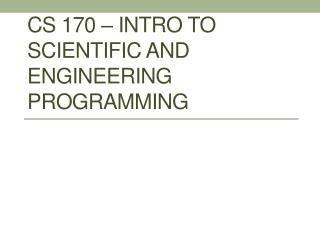DownloadDownload PresentationCS 170 – Intro to Scientific and engineering Programming

# CS 170 – Intro to Scientific and engineering Programming

Download Presentation## CS 170 – Intro to Scientific and engineering Programming

- - - - - - - - - - - - - - - - - - - - - - - - - - - E N D - - - - - - - - - - - - - - - - - - - - - - - - - - -
##### Presentation Transcript

1. CS 170 – Intro to Scientific and engineering Programming

2. Structures Data types encountered so far • numeric arrays, called double arrays • character arrays, called chararrays • container arrays, called cell arrays Today we cover structure arrays, called struct arrays. Like cell arrays, structure arrays allow you to group different data types together. Example: create a 1-by-1 structure with 3 fields >> student.Name = ’Fred Smith’; >> student.SID = 12345678; >> student.Scores = [62 78 93 61]; The variable student is created. It is a structure containing 3 fields.

3. class, size, fieldnames, isfield • Recall example >> student.Name = ’Fred Smith’; >> student.SID = 12345678; >> student.Scores = [62 78 93 61]; • Student is a 1-by-1 struct, with 3 fields, whose names are ’Name’ and ’SID’ and ’Scores’ >> size(student) >> class(student) >> fieldnames(student) >> isfield(student,’Name’) >> isfield(student,’Names’)

4. Accessing fields • Access the contents of the fields simply by typing VariableName.FieldName • So, in this case, we can do >> student.Name >> student.SID >> student.Scores • student.Scores is 1-by-4 double array. Everything works. For example, access its 1st element. >> student.Scores(1) • Or, access its last element. >> student.Scores(end)

5. Accessing fields (continued) • Can also assign as you would expect. Change the 3rd score to 99. >> student.Scores(3) = 99;

6. Structure Arrays How about using a struct to keep track of CS 170 students? But there are 22 of you. We need a structarray • with 22 elements • each with fields ’Name’, ’SID’ and ’Scores’. So far, every object in Matlab can be an array (not just scalar). Same holds for struct Can construct this by just declaring the the 2nd entry, and then the 3rd entry, and so on…

7. Growing the struct Add the 2nd student. Just assign, using the index… >> student(2).Name = ’William Hung’; >> student(2).SID = 10308090; >> student(2).Scores = [20 40 90 80]; Analogy: This works for all Matlab arrays. You can “grow” them simply by assigning beyond their current size. >> A = 1.3; >> A(2) %error, A only has 1 element >> A(2) = 4.7 % A is now 1-by-2 >> A(5) = 26 %A is now 1-by-5

8. Growing the struct (continued) Add the 3rd student. Just assign, using the index… >> student(3).Name = ’N. Coughlin’; >> student(3).SID = 22334455; >> student(3).Scores = [90 95 90 92]; Check that student is a struct array >> size(student) >> student >> whos

9. Accessing a field of Struct Array Recall that student is 1-by-3 struct array. One of its fields is named ’Scores’. In each case below, the expression is a 1-by-4 double array student(1).Scores student(2).Scores student(3).Scores What is student.Scores by itself?

10. Comma-separated Lists By itself, student.Scores is literally interpreted as Student(1).Scores , Student(2).Scores , Student(3).Scores 1-by-4 array 1-by-4 array 1-by-4 array comma comma Explicitly, based on the data, it is literally equal to [62 78 99 61] , [20 40 90 80] , [90 95 90 92] So, a field reference of a struct array represents a comma-separated list of the elements. A comma-separated list is not an object class (like double, char, cell or struct), but it can be used effectively in expressions…

11. Student.Scores vs [Student.Scores] Student.Scores actually represents [62 78 99 61] , [20 40 90 80] , [90 95 90 92] which is 3 arrays (all happen to be 1-by-4) separated by commas Recall that [[62 78 99 61] , [20 40 90 80] , [90 95 90 92]] is a 1-by-12 array (note the extra square brackets). Therefore, [student.Scores] is simply the 1-by-12 array [62 78 99 61 20 40 90 80 90 95 90 92]

12. Application of RESHAPE [Student.Scores] is a 1-by-12 array of test scores, [s1t1 s1t2 s1t3 s1t4 s2t1 s2t2 s2t3 s2t4 s3t1 s3t2 s3t3 s3t4] • The first 4 elements are the test scores of student 1 • The next 4 elements are the test scores of student 2 • The next 4 elements are the test scores of student 3 It would be nice to have a 3-by-4 or 4-by-3 array of the scores. The 4-by-3 case is “easier” since the order in memory is the same. The 3-by-4 case is “hard”, since the order in memory is different. Students Tests Tests Students

13. RESHAPE will work Try reshape([Student.Scores],[4 3]) To get the 3-by-4 case, you simply need to transpose the 4-by-3 case, so its not much harder (but Matlab shuffles everything in memory) reshape([Student.Scores],[4 3])’

14. Questions??